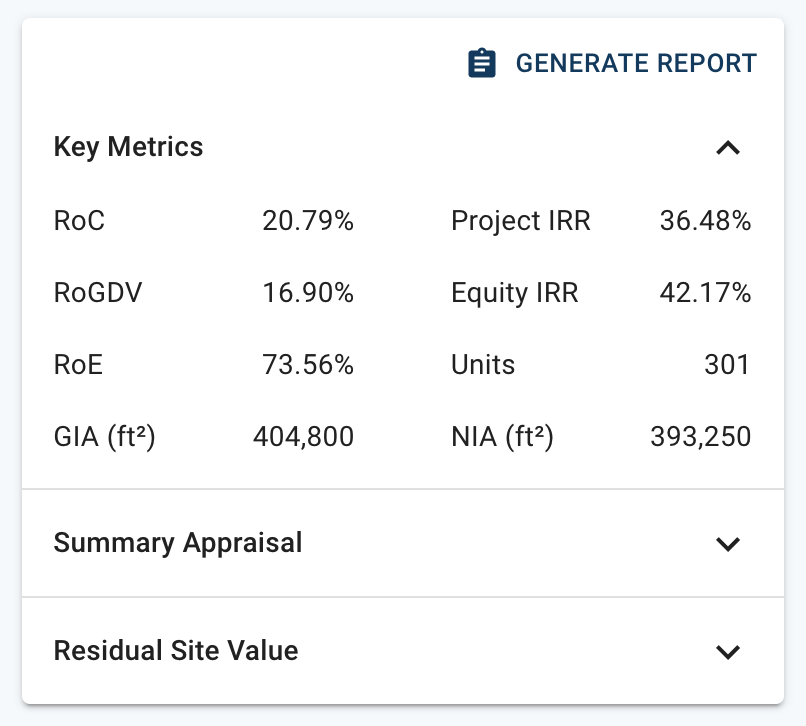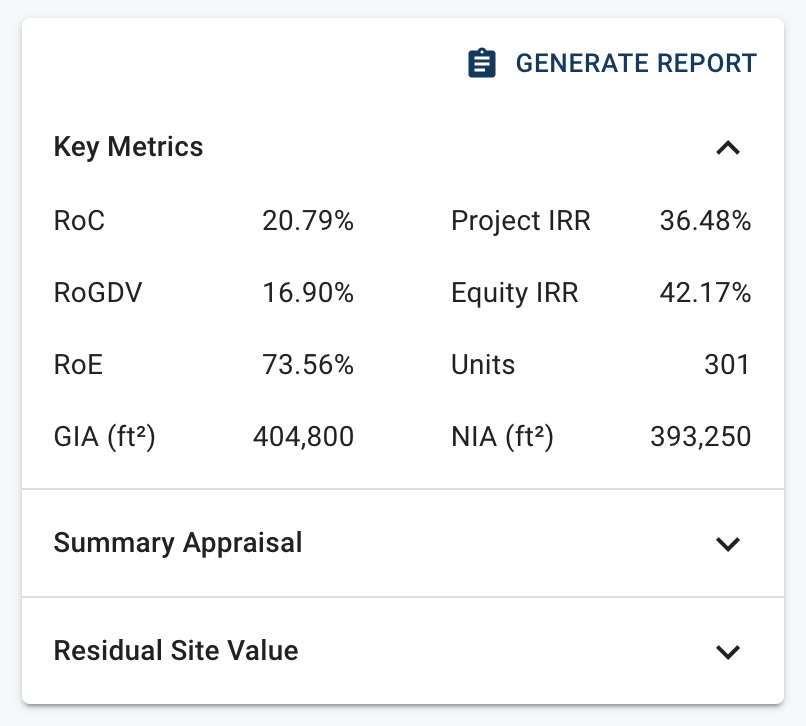Aprao DefinitionsThis article will guide you through the key metrics we use in Aprao, and will give some explanation as to how they are calculated.

On the right-hand side of Aprao, you can always see our "Key Metrics" panel, which is designed to give you a high-level view of the important information for your project. Whenever you make a change to your financials, the Key Metrics will update in real-time, so that you can make quicker and smarter decisions backed by these metrics.Let's break it down one-by-one, and explain them in a bit more detail.

RoC = Return on Cost

This is sometimes also referred to as Profit on Cost and is calculated simply as the total amount of profit (or return) divided by the cost and expressed as a percentage. This is useful in making sure your cost is not too high for the profit that is being generated. As a rule of thumb, a RoC of 18-25% is typical for development projects.

RoGDV = Return on GDV

This is sometimes referred to as Return on Cost and is calculated by taking the total profit (also known as return), divided by the GDV (gross development value) and then expressed as a percentage. This is useful to understand how your profit is relative to the completed value of a development project.

RoE = Return on Equity

This metric is designed to look at the financial performance of the equity going into a development project. This usually comes from the developer and of all the money in a development project, tends to run the highest risk. RoE is calculated by taking the return (or profit) and dividing it by the equity contributed to the project.

The higher the RoE, the more efficient the deal is on the equity going into the project.

Project IRR = Project Internal Rate of Return

This is the return of the development, based on the positive cash flow balance over time. This is a complex one to calculate yourself, but Aprao makes it nice and easy. The best way to think about this is by picturing two developments, both making \$1m profit. The project IRR assumes no finance on the project.

Equity IRR = Equity Internal Rate of Return

This is based on the same principles as the Project IRR but rather than being calculated on the net cash flow of the project, it is calculated on the equity contribution for the project. Therefore, the Equity IRR takes into account the development finance.

Units

This is the count of units to be sold in the completed development. This is a combination of residential and commercial units. For example, if a development has 40 residential apartments and 5 commercial units, the total unit count would be 45.

If you include income or other revenue, this will not be counted. For example, if you had 5 parking spaces to sell, these would not be included in the unit count.

NIA = Net Internal Area

This is the area within the units (residential or commercial) which will be sold. This is often the same as the NSA (net saleable area). The NIA excludes areas within the development which cannot be sold - such as internal staircases, lift areas, hallways and common areas. The NIA is used for the sales areas within Aprao.

GIA = Gross Internal Area

This is the total internal area of the development including areas which cannot be sold such as internal staircases, lift areas, hallways and common areas. The GIA is used in Aprao as the area from which the build cost is calculated.

A quick note on NIA and GIA

You can set a net to gross percentage in Aprao. For example, if you are building a development of apartments, typically the gross area is about 20% larger than the NIA. This can either be entered as a percentage, or - if the GIA is known - it can be entered as an area and this is then also displayed as a %.

We're here to help.

Not sure on anything above?

No worries, just reach out to support@aprao.com and our friendly team will be happy to help!